# Modulation of Oscillations

## Modulation of Oscillations

variation of the amplitude, frequency, or phase of oscillations that follows a specific pattern and is slow compared to the period of the oscillations; such modulation is correspondingly called amplitude modulation, frequency modulation, or phase modulation (Figure 1). In any method of modulation of oscillations, the rate of change of the amplitude, frequency, or phase must be slow enough so that the modulated parameter remains almost unchanged during each oscillation period.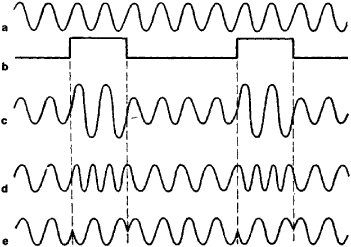Figure 1. Diagram of modulated oscillations: (a) unmodulated oscillation, (b) modulating signal, (c) amplitude-modulated oscillation, (d) frequency-modulated oscillation, (e) phase-modulated oscillation

Modulation of oscillations is used to transmit information by means of electromagnetic waves in the radio-frequency or optical regions. The signal carrier in this case is sinusoidal electrical oscillations of a high frequency ω(the carrier frequency). The amplitude, frequency, or phase (and, in the case of light, the polarization) is modulated by the signal being transmitted.

In the simplest case where the amplitude A is modulated by a sinusoidal signal, the modulated oscillation, which is shown in Figure 2, may be written in the form

(1) x = A0(1 + M sin Ωt) sin (ωt + ϕ)

Here A ω and co are, respectively, the amplitude and frequency of the original oscillation, Ωis the modulating frequency, and the quantity m, the depth of modulation, describes the degree of variation of amplitude: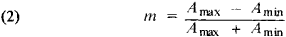The frequency of modulation Ω describes the rate of variation of the amplitude of the oscillations. This frequency must be many times smaller than the carrier frequency ω. The modulated oscillation is no longer sinusoidal. An amplitude-modulated oscillation is the sum of three sinusoidal oscillations of frequencies, ω ω + Ω , and ω − Ω. The oscillation with frequency ω is called the carrier. Its amplitude is equal to the amplitude of the original oscillation A0. The other two frequencies are called the side frequencies. Amplitude of each is equal to mA0/2.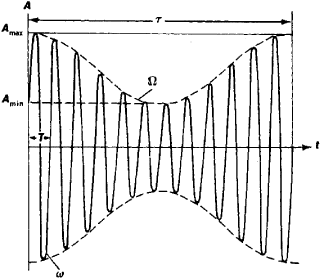Figure 2. Amplitude modulation by a sinusoidal signal: (ω) carrier frequency, (Ω) frequency of the modulating oscillations, (Amax) and (Amax) maximum and minimum values of amplitude, respectively

Thus, any radio station that is operating in the amplitude-modulation mode radiates not just one frequency but an entire set (spectrum) of frequencies. In the simplest case, where the oscillations are being modulated by a sinusoidal signal, the spectrum contains only three components, a carrier and two side frequencies. When the modulating signal is not sinusoidal but rather more complex, then instead of two side frequencies in the modulated oscillation there will be two sidebands, whose frequency composition is determined by the frequency composition of the modulating signal. As a result, each station occupies a particular frequency band. To avoid interference, the carrier frequencies of different stations must be separated from one another by a spacing that is greater than the sum of the sidebands. The width of a sideband depends on the nature of the transmitted signal: for radio broadcasting it is 10 kilohertz (kHz), and for television it is 6 megahertz (MHz). The separation between the carrier frequencies of different stations is chosen on the basis of these values. To achieve an amplitude-modulated oscillation, the carrier-frequency oscillation of frequency co and the modulating signal at a frequency Ω are fed into a special device called a modulator.

In the case of frequency modulation by a sinusoidal signal, the frequency is varied according to the law

(3) ω = ω0 + Δω cos Ωt

where cos ftt is the modulating signal and Δωco is the frequency deviation. In frequency modulation the frequency band of the modulated oscillation is a function of the quantity β = Δβ/Ω, which is called the frequency-modulation index. When β ≪ 1, the following approximate expression is valid:

(4) xA0 (sin ωt + β sin Ωt cos Ωt

In this case the frequency-modulated oscillation, like an amplitude-modulated oscillation, consists of a carrier frequency ω and two sideband frequencies ω + Ω and ω − Ω Consequently, when β is small the frequency bands occupied by amplitude-modulated and frequency-modulated signals are the same. When the index β is large, the spectrum of the side frequencies is considerably larger. In addition to oscillations of frequencies ω ± ft,there are oscillations of frequencies ω ± 2Ω, ω ±3Ω, and so on. The total bandwidth occupied by a frequency-modulated oscillation with the deviation Aco and a modulating frequency Ω (within an accuracy sufficient for practical purposes) can be considered to be equal to 2Δω + 2Ω. This band is always wider than in the case of amplitude modulation.

The advantage of frequency modulation over amplitude modulation in communications technology is the high noise immunity. This property of frequency modulation is exhibited when β ≫ 1—that is, when the frequency band occupied by the frequency-modulated signal is many times greater than 2ω. Therefore, frequency-modulated oscillations are used for high-quality transmission of signals in the ultrashort-wavelength range, where each radio station is separated by a frequency band 15–20 times greater than in the medium- and short-wavelength ranges, in which amplitude-modulated radio stations operate. Frequency modulation is also used to transmit the sound accompanying television programs. Frequency-modulated oscillations may be produced by varying the frequency of a driving oscillator.

In phase modulation, the modulated oscillation has the form

(5) x = A0 sin (ω0 + Δϕ cos Ωt

If the modulating signal is sinusoidal, the shape and spectral composition of the modulated oscillations are the same in frequency and phase modulation. In the case of a nonsinusoidal modulating signal, the difference is pronounced.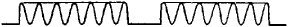The information carrier used in multichannel communications systems is not a harmonic oscillation but rather a periodic sequence of radio-frequency pulses, each of which consists of a train of high-frequency oscillations (Figure 3). A periodic sequence of such pulses is defined by four basic parameters: amplitude, repetition frequency, duration (width), and phase. Accordingly, there are four possible types of pulse modulation: pulse-amplitude, pulse-frequency, pulse-width, and pulse-position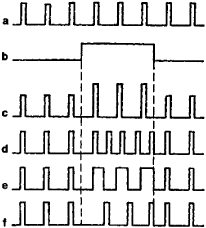Figure 4. Various forms of pulse modulation: (a) unmodulated sequence of radio-frequency pulses, (b) transmitted signal, (c) pulse-amplitude modulation, (d) pulse-frequency modulation, (e) pulse-width modulation, (f) pulse-position modulation

(Figure 4). Pulse modulation has a higher noise immunity than the modulation of a continuous sinusoidal carrier, but the frequency band occupied by a radio station transmitting with pulse modulation is many times wider than in the case of amplitude modulation.

### REFERENCES

Kharkevich, A. A. Osnovy radiotekhniki, part 1. Moscow, 1962.
Goldman, S. Garmonicheskii analiz, moduliatsiia i shumy. Moscow, 1951. (Translated from English.)
Rytov, S. M. “Modulirovannye kolebaniia i volny.” Tr. Fizicheskogo in-ta AN SSSR, 1940, vol. 2, fasc. 1.

V. N. PARYGIN

Site: Follow: Share:
Open / Close